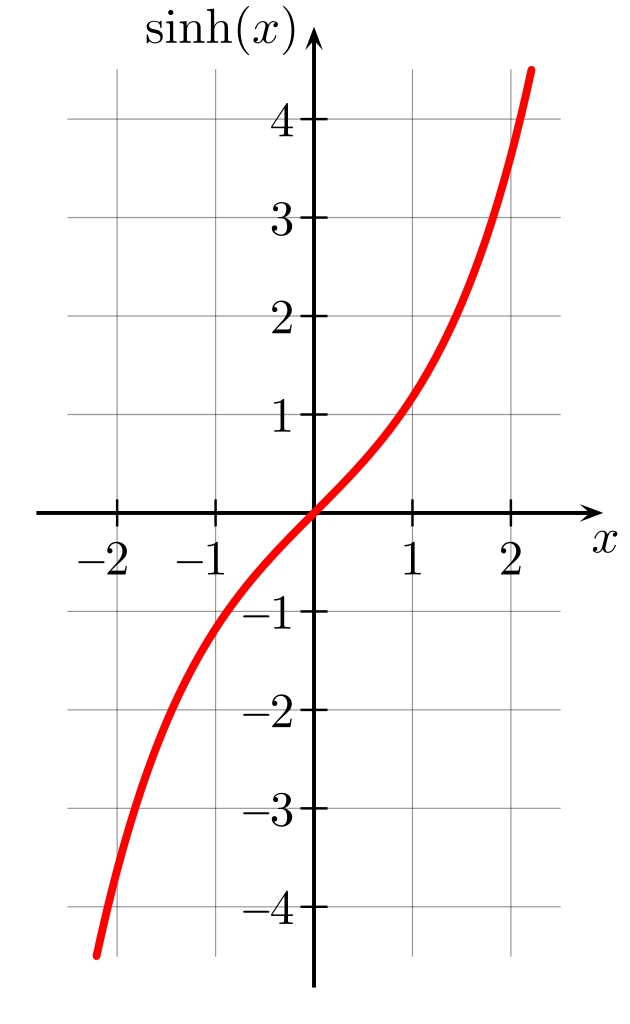# Sinh Calculator

Created by Anna Szczepanek, PhD
Reviewed by Luis Hoyos
Last updated: Jun 05, 2023

Topics range from really basic (what sinh x actually is and what are the basic sinh and cosh identities) to more slightly advanced (the inverse hyperbolic sine and the derivative of sinh).

As a bonus, we'll discuss how to put hyperbolic sine in a calculator (we mean a really basic calculator) should it ever happen you don't have access to Omni and its hyperbolic sine calculator.

## What is sinh x in math?

Sinh is a function in math called hyperbolic sine. It is defined as

$\small \sinh x = \frac{1}{2}(\mathrm{e}^x - \mathrm{e}^{-x})$

But what does it have to do with sine? we hear you ask. To see the connection, we must introduce the twin of sinh, that is the cosh (also known as hyperbolic cosine), defined as

$\small \cosh x = \frac{1}{2}(\mathrm{e}^x + \mathrm{e}^{-x})$

Now if we plot points with coordinates ($\cosh x$, $\sinh x$) in the standard Cartesian coordinate system, we obtain a hyperbola, as you can see in the picture below. (More precisely, we'll obtain only the right-hand side part of the hyperbola.)

Recall that when we plot the points ($\cos x$, $\sin x$), they form a circle (the unit circle). And the similarities do not end here! It turns out our hyperbolic sine and hyperbolic cosine satisfy certain formulae that are very similar, or sometimes even identical, as those that we know for the good ol' (non-hyperbolic) sine and cosine. Namely, we have the double-angle formula

$\small \sinh 2t=2\sinh t\cosh t$

and

$\small\cosh ^{2}x-\sinh ^{2}x=1$

which is an analogue of the Pythagorean trigonometric identity $\cos ^{2}x+\sin ^{2}x=1$.

## Graph and properties of sinh

Let us plot the graph of the sinh function.Looking at the plot, we can discover some properties of the hyperbolic sine:

• sinh is an odd function, i.e., $\sinh(-x) = -\sinh(x)$;
• sinh is increasing;
• $\sinh(0) = 0$;
• sinh is not periodic;
• sinh is not bounded; and
• sinh is a bijection, so it has an inverse.

So how can you calculate the inverse of sinh? Let's move on to the next section.

## What is the inverse of hyperbolic sine?

The inverse function of sinh is denoted by arsinh. The formula for arsinh reads:

$\small \mathrm{arsinh}\ x = \ln \left(x + \sqrt{x^2 + 1}\right)$

It involves logarithms, which is what we expected since the definition of sinh involves exponentials.

Be careful not to confuse the inverse function of sinh with the multiplicative inverse, that is, with the function mapping $x$ to $1/\sinh x$. This function has its own name: its called csch (Not a very intuitive name, we admit...):

$\small \text{csch}\ x = \frac{1}{\sinh x} = \frac{2}{(e^x - e^{-x})}$

if $x\! \not =\! 0$.

## How to use this sinh calculator?

Omni's hyperbolic sine calculator is very straightforward to use: just enter the argument x, and the value of sinh(x) will appear immediately!

And you can calculate inverse sinh as well! Just fill in the field sinh(x), and the value that appears as x is exactly the value of the inverse function.

And this is not all! If you hit the advanced mode button beneath the calculator, you'll be able to see the values of several other hyperbolic functions, in particular of the derivative of sinh - it hides under the name of cosh ;)

## Similar Omni calculators

Since hyperbolic functions like to pop up in calculations related to various domains, it's important you familiarize yourself with them a bit! Once you're done with the sinh calculator, do not hesitate to visit the following tools:

## FAQ

### How do I put hyperbolic sine in a calculator?

To compute sinh(x) with a basic calculator:

1. Make sure your calculator can perform exponentiation. If not, search for an online exponentiation calculator (or go straight to an online sinh calculator).
2. Compute exp(x) and store the result (write it down or use the memory of your calculator).
3. Compute exp(-x) and store the result.
4. Compute exp(x) - exp(-x).
5. Divide the result by 2. That's it; well done!

### What is the derivative of sinh?

The derivative of sinh is cosh, that is, the hyperbolic cosine, defined as cosh(x) = (exp(x) + exp(-x))/2. You can easily remember this fact by recalling that this is exactly what happens with standard sine and cosine functions!

### How do I calculate sinh 1 given cosh 1?

To compute sinh 1 given cosh 1

1. Use the cosh²x - sinh²x = 1 identity. It gives us the following:
sinh²(1) = cosh²(1) - 1
2. Now you can perform a simple subtraction:
sinh²(1) = 1.543² - 1 = 2.381 - 1 = 1.381
3. Then, take the square root of sinh²(1):
sinh(1) = 1.175.
Anna Szczepanek, PhDx
sinh(x)
For other hyperbolic functions, hit the Advanced mode button below.
People also viewed…

### Car vs. Bike

Everyone knows biking is fantastic, but only this Car vs. Bike Calculator turns biking hours into trees! 🌳

### Circle measurements

Our circle measurements calculator helps to calculate the radius, diameter, circumference and area of a circle.

### Round to the nearest cent

Use this tool to calculate the rounding to the nearest cent of any number or money amount you want.